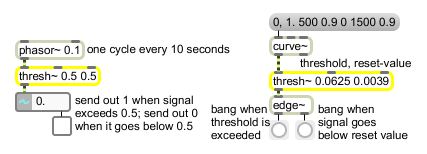# thresh~

Detect signal above a set level

## Description

thresh~ initially outputs a signal of 0. It outputs a signal of 1 when the input is equal or greater than a set "high" threshold, until it goes below the reset or "low" threshold, at which point the 0 signal is output again. If the low and high thresholds are the same, a reset occurs when the signal is less than the threshold value. Otherwise, a reset occurs when the signal is less than or equal to the low threshold. The low and high thresholds can be specified as arguments, via floats in the middle and right inlets, or as signals in the middle and right inlets.

## Arguments

Name Type Opt Description
low/reset-threshold and high/set-threshold float The first argument specifies the reset or low threshold level. If no argument is present, the reset level is 0. The second argument specifies the set or high threshold level. If no argument is present, the set level is 0.

If only one argument is present, it specifies the reset level, and the set level is 0.

## Messages

 float reset/set-threshold [float] In middle inlet: Sets the lower ("reset") threshold level for the input signal. When a sample in the input signal is greater than or equal to the upper ("set") level, thresh~ sends out a signal of 1 until a sample in the input signal is less than or equal to this reset level. In right inlet: Sets the upper ("set") threshold level for the input signal. When the input is equal to or greater than this value, thresh~ sends out a signal of 1. signal In left inlet: A signal whose level you want to detect.

## Output

signal: When a sample in the input signal is greater than or equal to the upper threshold level, the output is 1. The output continues to be 1 until a sample in the input signal is equal to or less than the reset level. If the set level and the reset level are the same, the output is 1 until a sample in the input signal is less than the reset level.

## ExamplesDetect when signal exceeds a certain level